How Cheenta works to ensure student success?
Explore the Back-Story

# Continuous Function: TIFR 2017 Problem 10# Understand the problem

There exists a non-negative continuous function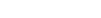such that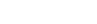as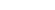(a) TRUE (b) FALSE

Do you really need a hint? Try it first!

"Hint 1" : Rather I want to say that it is a comment on the question that here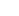does not mean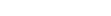(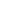times). Here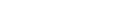(times). Now do you want to think again with this disclosure? "Hint 2" : Make two cases Case 1: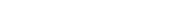[Observe that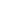is non negative function] Case 2: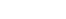for some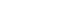Now prove for each case thatas. So, by the last statement you have guessed the validity of the statement . It is a false statement!! In the next two cases, we will basically prove two cases. "Hint 3" : Case 1:  If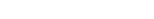then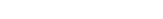So,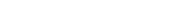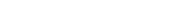So,as"Hint 4" : Case 2:  Supposefor someNow, as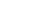is continuous function We have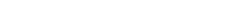for some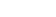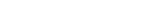then we have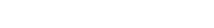[If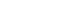or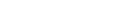[If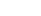] or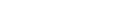[If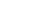] In either case ,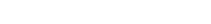So, the statement is false.

# Similar Problems

The higher mathematics program caters to advanced college and university students. It is useful for I.S.I. M.Math Entrance, GRE Math Subject Test, TIFR Ph.D. Entrance, I.I.T. JAM. The program is problem driven. We work with candidates who have a deep love for mathematics. This program is also useful for adults continuing who wish to rediscover the world of mathematics.

# Understand the problem

There exists a non-negative continuous functionsuch thatas(a) TRUE (b) FALSE

Do you really need a hint? Try it first!

"Hint 1" : Rather I want to say that it is a comment on the question that heredoes not mean(times). Here(times). Now do you want to think again with this disclosure? "Hint 2" : Make two cases Case 1:[Observe thatis non negative function] Case 2:for someNow prove for each case thatas. So, by the last statement you have guessed the validity of the statement . It is a false statement!! In the next two cases, we will basically prove two cases. "Hint 3" : Case 1:  IfthenSo,So,as"Hint 4" : Case 2:  Supposefor someNow, asis continuous function We havefor somethen we have[Ifor[If] or[If] In either case ,So, the statement is false.

# Similar Problems

The higher mathematics program caters to advanced college and university students. It is useful for I.S.I. M.Math Entrance, GRE Math Subject Test, TIFR Ph.D. Entrance, I.I.T. JAM. The program is problem driven. We work with candidates who have a deep love for mathematics. This program is also useful for adults continuing who wish to rediscover the world of mathematics.

This site uses Akismet to reduce spam. Learn how your comment data is processed.

### Knowledge Partner